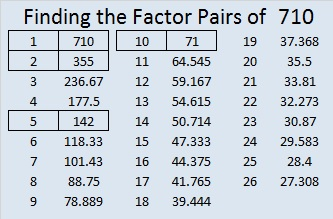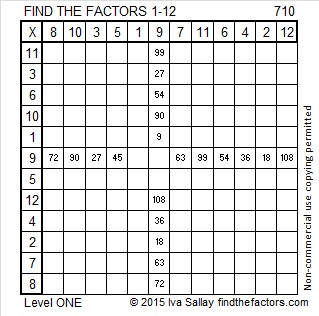# 710 and Level 1

• 710 is a composite number.
• Prime factorization: 710 = 2 x 5 x 71
• The exponents in the prime factorization are 1, 1, and 1. Adding one to each and multiplying we get (1 + 1)(1 + 1)(1 + 1) = 2 x 2 x 2 = 8. Therefore 710 has exactly 8 factors.
• Factors of 710: 1, 2, 5, 10, 71, 142, 355, 710
• Factor pairs: 710 = 1 x 710, 2 x 355, 5 x 142, or 10 x 71
• 710 has no square factors that allow its square root to be simplified. √710 ≈ 26.645825.Here is today’s puzzle:Print the puzzles or type the solution on this excel file: 12 Factors 2015-12-14

———————————————————————————

Here are few more facts about 710:

710 can be written as the sum of consecutive numbers several ways:

• 176 + 177 + 178 + 179 = 710; that’s four consecutive numbers.
• 140 + 141 + 142 + 143 + 144 = 710; that’s five consecutive numbers.
• 26 + 27 + 28 + 29 + 30 + 31 + 32 + 33 + 34 + 35 + 36 + 37 + 38 + 39 + 40 + 41 + 42 + 43 + 44 + 45 = 710; that’s 20 consecutive numbers.

710 is the sum of the twenty prime numbers from 3 to 73.

Because 5 is one of its factors, 710 is the hypotenuse of Pythagorean triple 426-568-710. What is the greatest common factor of those three numbers?

710 is palindrome 868 in BASE 9; note that 8(81) + 6(9) + 8(1) = 710.

———————————————————————————This site uses Akismet to reduce spam. Learn how your comment data is processed.